# Power Tips: How to use Nyquist plots to assess system stability

Article written by Manjing Xie

A Bode plot is a very popular way to determine a dynamic system’s stability. However, there are times, when a Bode plot is not a straightforward stability indicator.

Figure 1 shows a Bode plot of TI’s TPS40425synchronous buck converter with. In this application, a π filter is used at the output of the buck converter.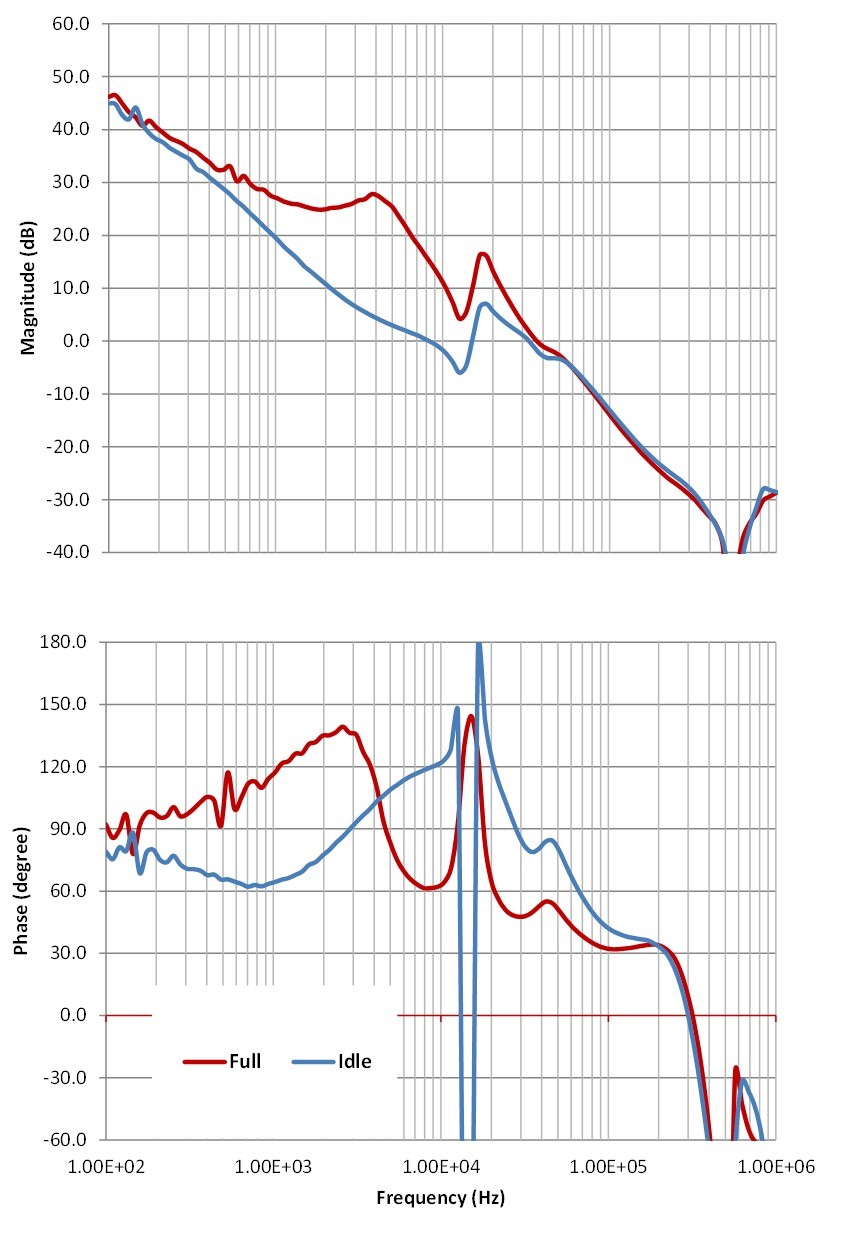Figure 1: Bode plot of a buck converter with an output π filter

Because the ferrite bead used in the π filter has varying inductance over the load current, the Bode plots measured at different load conditions vary dramatically. At idling, the Bode plot measured has multiple 0db gain crossover. It is difficult to apply the phase margin and gain margin criteria in this case. Instead, I used Nyquist stability criterion.

The Nyquist stability criterion looks at the Nyquist plot of open-loop systems in Cartesian coordinates, with s = jω. Assuming that the open-loop system transfer function is F(s), the Nyquist plot is a plot of the transfer function of F (jω), with ω from -∞ to +∞. Stability is determined by looking at the number of encirclements of the point at (-1,0j). If the number of counterclockwise encirclements of (-1,0j) by F(s) equals the right-half-plane poles of F(s), then the system is stable. In this example, if a buck converter does not have right-half-plane poles, the number of encirclements of (-1,0j) indicates the stability.

You can derive a Nyquist plot from the measured Bode plot. I save the data first. The analyzer I use provides the data with magnitude in decibels and phase in degrees, as shown in Figure 2. Different frequency analyzers provide different formats. There are frequency analyzers that provide the data in complex numbers.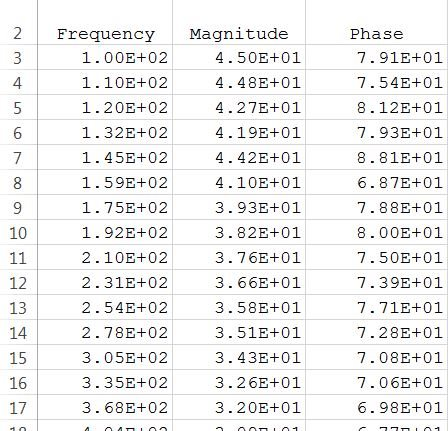Figure 2: Data saved by a frequency analyzer from a Bode-plot measurement

Equation 1 converts magnitude and phase into complex numbers: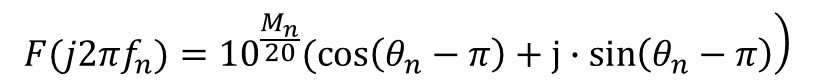(1)

Where Mn is the magnitude and θn is the phase of measured F(s) with s = j2πfn.

After applying Equation 1, I plotted the complex numbers in Cartesian coordinates. The plot show in Figure 3 is of frequencies from 100Hz to 1MHz. It is a good approximation of plot from 0Hz to +∞Hz. The plot from -∞Hz to 0Hz is the plot from 100Hz to 1MHz mirrored horizontally in Cartesian coordinates. I added the part from plot from -∞Hz to 0Hz to Figure 3 and formed Figure 4.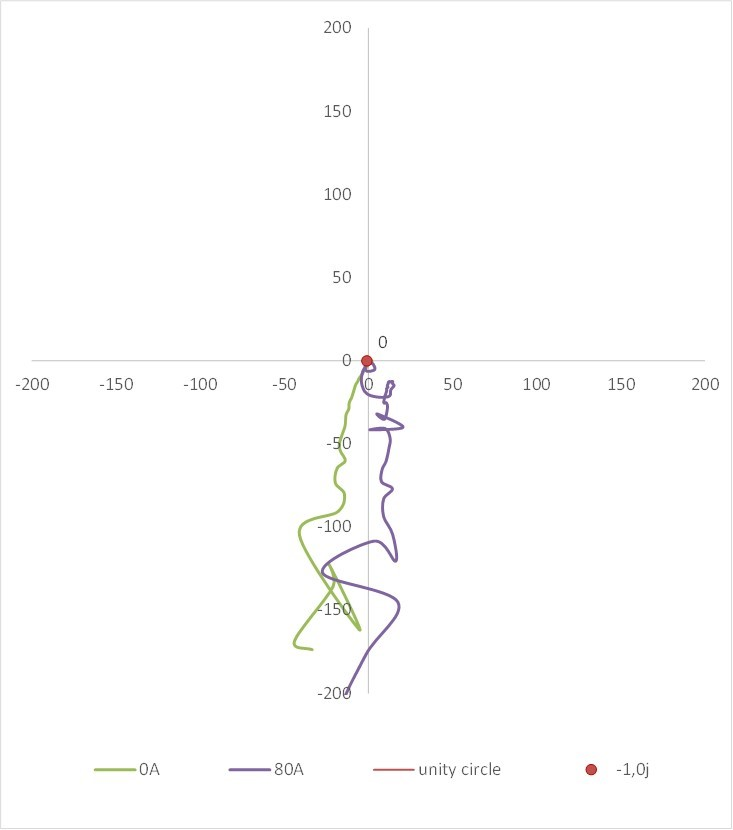Figure 3: A Nyquist plot derived from a Bode plot of frequencies from 100Hz to 1MHz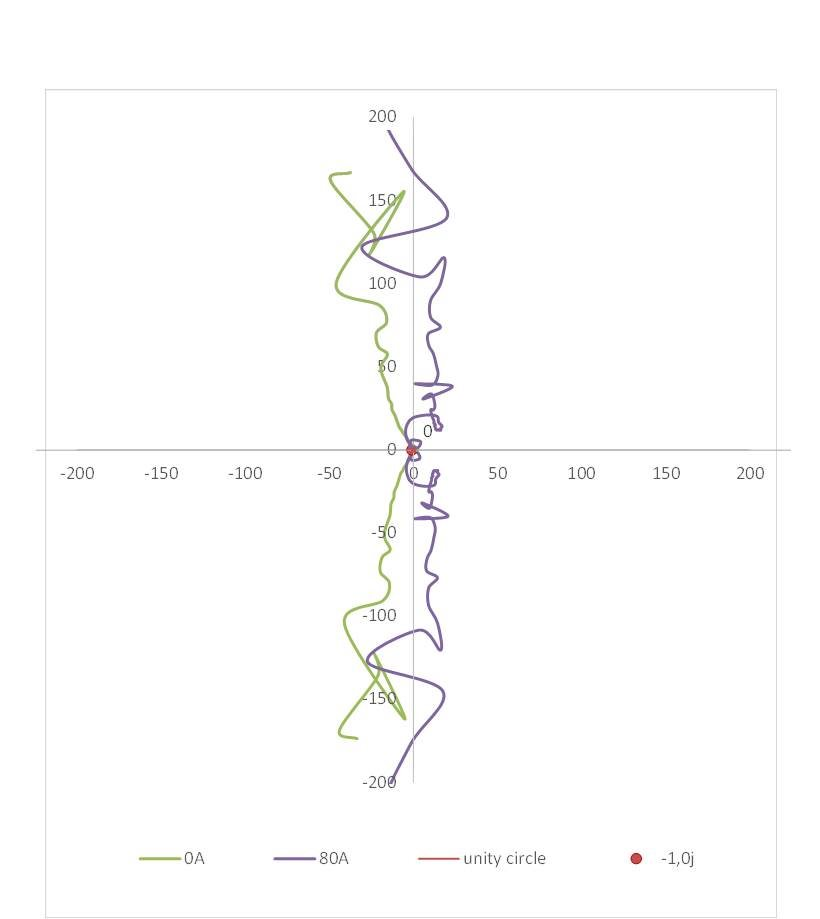Figure 4: A Nyquist plot derived from a Bode plot from -1MHz to -100Hz and 100Hz to +1MHz

It is the path around the unity gain circle that is most relevant to the system stability.  I zoomed into the area close to the unity gain circle. Since this system is of a voltage-mode buck converter, I know the DC gain is over 90dB, with a phase starting from 0 degrees. I can approximate the plot at lower frequencies in the complete Nyquist plot, as shown in Figure 5.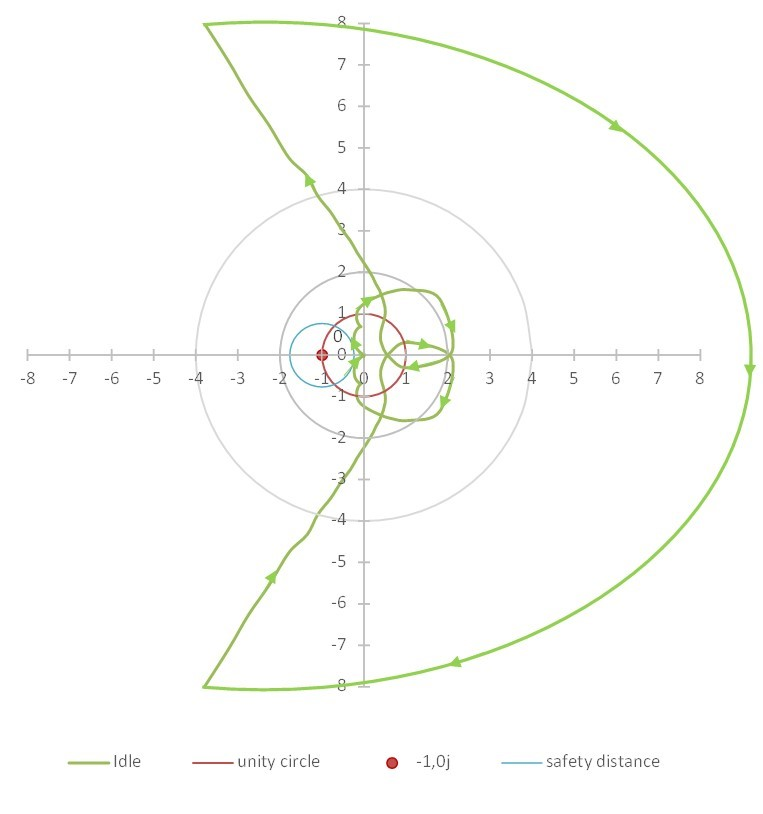Figure 5: Approximate Nyquist plot from -∞Hz to +∞Hz when the system is idling

Following the arrows from -∞Hz, you can see that the Nyquist plot does not encircle the (-1,0j) point. The system is stable. I plotted a blue circle with a radius of 0.766 and (-1,j0) as the center. If the Nyquist plot does not enter this blue circle, the phase margin is greater than 45 degrees and the gain margin should be greater than 12dB.

Following the same procedure, Figure 6 shows a Nyquist plot at full load. Following the arrows, you can see that the Nyquist plot doesn’t encircle the (-1,0j) point either. And this Nyquist plot is outside of the safety circle, as specified earlier.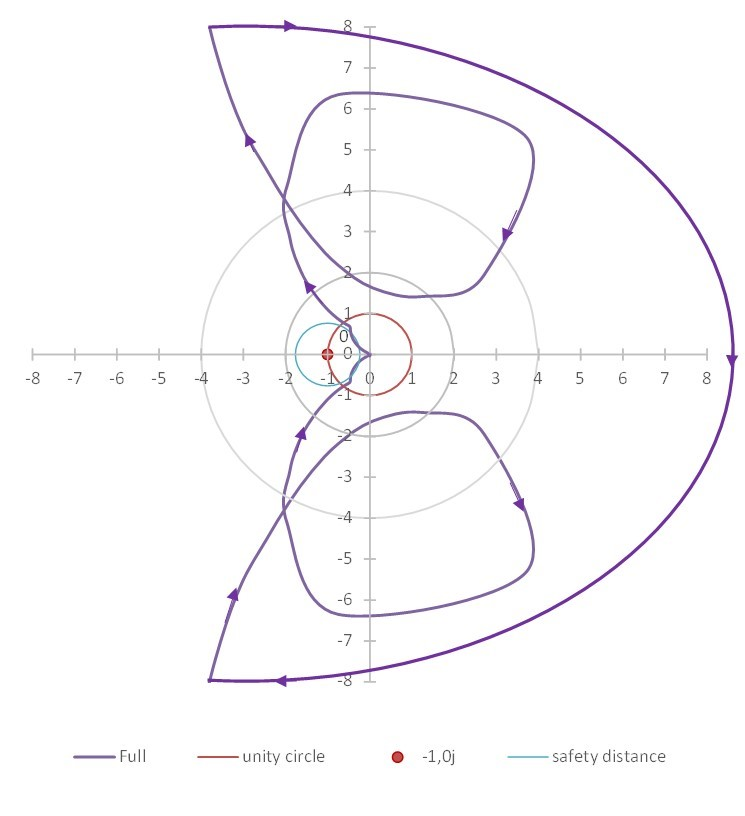Figure 6: Approximate Nyquist plot from -∞Hz to +∞Hz when the system is at full load

When Bode plots fail to provide a straightforward indication of stability, consider using a Nyquist plot. I’ve shown you in this post how to convert measured Bode plots to a Nyquist plot and how to use Nyquist stability criterion to judge system stability.